# Payback Period and Discounted Payback Period

Please give an example that explains payback period and discounted payback period. How do we decide whether a project should be undertaken or not based on these methods?

This post was part of TyroCity discussion forum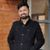Angel Paudel

The payback period can be defined as a length of the period required within which the investment/cost is fully recovered by the business/project. This factor plays an important role in determining whether a project should be undertaken or not. It doesn’t take into account the time value of money (TVM) but only accounts for the absolute value of cash flow (Rushinck, 1983). In the equation, it can be referred to as:

Payback period = Cost of project/investment (divided by) Annual Cash Inflow

Considering an example for payback period, let’s assume an organization invested NRs. 5,00,000 in a project. The organization projects to get a return of NRs. 1,25,000 per annum evenly throughout the project cycle. Considering that and using the formula as listed above, we get payback period to be 5,00,000/1,25,000 which equals to 4. That means the organization’s payback period is 4 yrs.

Discounted payback period is same as payback period with a slight difference that it also takes in account the time value of money (TVM). This form of calculation helps also calculate the actual risk involved in the project while considering the time factor to take in account the value of money (Bhandari, 1989). It still fails to consider the cash flow that occurs after the payback is complete even though it’s considered to be a better practice to follow this over payback period. It can be expressed in the equation as:

Discounted payback period = Actual Cash flow / (1+i) ^ n

Considering an example to calculate the discounted payback period, let’s consider that an organization made an investment of NRs. 5,00,000 while it gets 2,00,000 cash flow every year. Considering the discount rate is 12%, the calculation process for the same is as listed below (the values in the bracket are to denote that they represent a negative number):

Year (n) Cash flow of the project (CF) Present value (PV) = [1/(1+i)^n] Discounted cash flow (CF*PV) Cumulative value
0 (500000) 1 (500000) (500000)
1 200000 0.89 178000 (322000)
2 200000 0.8 160000 (162000)
3 200000 0.71 142000 (20000)
4 200000 0.64 128000 108000

So, the discounted payback period is 3+ {20000 / (20000+108000)} = 3.16 yrs.

To decide if a project is to be taken or not, the best approach for this would be to look at the projected time horizon that the business intends to hit profitability. If we consider the payback period to be 5 yrs. and the project paid off for it within 4.5 yrs. then it’s considered that the project does generate profit within the set timeframe for the business and should be taken. However, if the project payback period is 6 yrs. but the business wants to hit profitability within 5 yrs. then the project isn’t good and shouldn’t be taken.

Thus, if the payback is positive which simply means that the project payback is less than what the business expects to enter profitability by than that’s a good signal that the project should be considered. The larger the difference (towards a lesser number), the favorable it is and should be considered but if that goes even a fraction above the company’s set target, it’s worth letting it go and not considering.

References

Bhandari, S. (1989). Discounted Payback Period – A Viable Complement to Net Present Value for Projects with Conventional Cash Flows. The Journal Of Cost Analysis, 7 (1), 43-44.

Rushinck, A. (1983). Capital Budgeting Techniques, The Payback Period, The Net Present Value, The Internal Rate of Return and their Computer Applications. Managerial Finance , 9 (1), 11-13.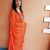Payback period (PBP) calculates the time required to recover initial investment made in a project in nominal sense. It is the time period when the cumulative cash inflows from a project equals the amount of initial cash outlay of the project (Moyer, McGuigan, Rao, & Kretlow, 2012). Discounted Payback Period (DPBP) also measures the time needed to recover the original costs of investment but it takes into consideration the time value of money unlike PBP. In DPBP, the cash flows are discounted at required rate of return (cost of capital) before calculating the period of payback.

Let us consider the following examples.

Project A:

Initial Investment = Rs. 120,000

Discount Rate = 10 %

Year Cash flow (CF) in Rs. Cumulative CF PV Factor @ 10% Discounted CF Cumulative Discounted CF
0 -120,000 -120,000 1 -120,000.00 -120,000.00
1 48,000 -72,000 0.9091 43,636.80 -76,363.20
2 50,000 -22,000 0.8264 41,320.00 -35,043.20
3 40,000 18,000 0.7513 30,052.00 -4,991.20
4 39,000 57,000 0.6830 26,637.00 21,645.80

Here,

PBP = Number of years before full recovery + ( Unrecovered Amount at the Start of Year/Cashflow During the Year)

= 2 + (22,000/40,000)
= 2.55 years

DPBP = Number of years before full recovery + ( Unrecovered Discounted Amount at the Start of Year/Discounted Cashflow During the Year)

= 3 + (4,991.20/26,637)
= 3.19 years

Project B:

Initial Investment = Rs. 50,000

Cost of Capital = 10 %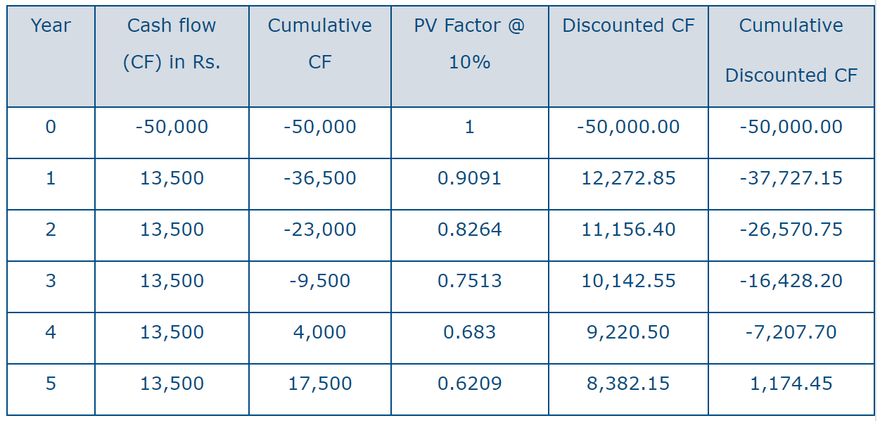Here,

PBP = Net Investment/Annual Cashflow

= 50,000/13,500
= 3.7 years

DPBP = Number of years before full recovery + ( Unrecovered Discounted Amount at the Start of Year/Discounted Cash flow During the Year)

= 4 + (7207.70/8382.15)
= 4. 85 years

Results:

Project Payback Period Discounted Payback Period
A 2.55 years 3.19 years
B 3.7 years 4.85 years

From the above result, we can see that the discounted payback period is higher than the payback period because it considers the discounted cash flow for determining the duration for recovering the initial investment of a project.

Decision Criteria

Although payback period is not an effective criteria to decide about the acceptance of a project, it is used at times because it is easy to calculate and understand. In case of independent projects, the management has a pre-determined standard payback period which is usually three or four years and the projects that have less payback period than the standard period are accepted. In case of mutually exclusive projects, the project with lower payback period is selected.

Let us assume the standard payback period for the organization is 4 years. If projects A and B are independent projects, both are selected on the criteria of payback period. Analyzing from the discounted payback period concept, project A is selected and B is rejected as the payback period of project B exceeds the standard set by management.

If these projects are mutually exclusive, project A is selected as its payback period is less than that of the project B.

References

Moyer, R. C., McGuigan, J. R., Rao, R., & Kretlow, W. J. (2012). Contemporary Financial Management (12th ed.). Oklahoma: Cengage Learning.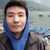In an enterprise, an entrepreneur should know the period for the return of the investment done. This information is very important to plan and start the business. The shorter the period of return the more beneficial as it reduces the risk factors. Let us look into two separate methods to calculate the period of return.

Pay Back Period (PBP)

PBP is thus simply the number of years that it will take the business to earn back the amount of initial investment or reach a breakeven point (BEP). This is calculated as total investment divided by the net cash flow of each year until BEP is maintained. For example an initial investment of Rs. 200,000 is done. The expected net cash inflow per year is expected to be Rs. 50,000 then using the formula of total investment divided by the net cash inflow per year we get 4 years.

PBP = Total initial investment / Annual net cash inflow

This means that the investment of Rs. 200,000 with annual net cash inflow of Rs. 50,000 the amount will be earned back in 4 years. This is a simple method that people from all background can calculate and can be used in simple terms. But this method has limitations as it does not consider the time value of money. Therefore a discounted pay back period is used for this purpose.

Discounted Pay Back Period

Discounted pay back period does the same thing as pay back period. It calculates the no of years for return of investment. The difference is that it deducts the expected net cash flow from the initial investment by converting it into the present value or year zero value (starting date of year one where investment is done). It does this because it considers the time value of money. Time value of money says that Rs. 100 today is more valuable than Rs. 100 one year later due to its earning capacity within that one year period. This is why the net cash inflow one year hence cannot be directly deducted from the initial investment but rather converted to the year zero value (discounted cash flow) and then deducted. "Under the discounted rate of return method a discounted rate is computed to determine the present value of the money to be recovered from the project during its future service life.” (Haglund, 1964)

Let us take Rs. 200,000 as initial investment and Rs. 50,000 as expected net cash inflow for the next 6 years. Let us assume the discounted rate to be 10% then the discounted cash flow will be calculated as follows.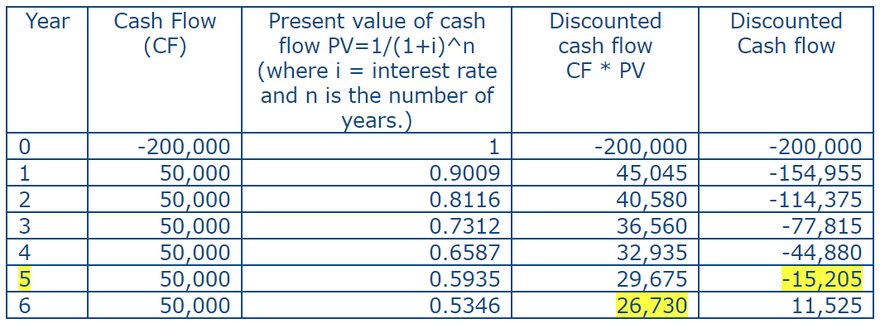Now the discounted pay back period will be

= 5+15205/26730

= 5.6 years.

Thus pay back period is before the expected number of years so it is good to accept the project. Less pay back period is better.

These examples have looked at constant expected cash flow annually. Things are a bit different for non-constant cash flow in which the process will be the same. In PBP however the cumulative cash flow are added until there is BEP.

The PBP and discounted PBP both calculate the years required for return on investment. As we can see discounted period is longer than simple pay back period but discounted period is more accurate.

Reference

Haglund, B. E. (1964). Discounted rate of return on capital investments. Journal of Accountancy, 84.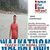Historically, the payback period method has been used as a formal method to evaluate project’s riskiness since positive cash flow deals how fast an investment can be recovered. Quite simply, the payback period method calculate the number of years required for positive cash flows to just equal the total initial investment (I) (Hoskins & Mumey, 1979). Hence, the method screens the projects on the basis of how long it takes for net receipts to equal investment. But it does not for saving after payback period generated by the project. A low value payback period is considered for the better project when choosing among mutually exclusive projects.

On the basis of way to compute, pay­back period method can be classified into two types

Simple Payback Period

Simple payback indicates the requirement time period to break even on an investment without considering time value of money. It doesn’t consider the cash flow amount after payback period.

It is compute as follows:

Simple payback Period (SP) = Initial Investment / Expected savings or Net cash inflow per period

• Easy to calculate
• It is interpret in terms of time.
• It doesn’t require any assumption about the project in terms of timing like useful life, interest rate etc.

• Fails to measure profitability i.e. no profit is made during the payback period.
• It ignores time value of money.
• It does not account for savings after the payback period.

Example: Simple payback periods from the given cash flows of project when MARR is 20%

End of period Net cash flow (Rs) Cumulative cash flow (Rs)
0 -25000 -25000
1 +8000 -17000
2 +8000 -9000
3 +8000 -1000
4 +8000 +7000
5 +13000 +20000

Here, cumulative cash flow turns to positive in period 4. Therefore, payback periods lies between period 3 and 4. By interpolating we get payback period is
= 3 + 1000/8000
= 3.125 periods.

Discounted Payback period

This method includes time value money for determining payback period. Hence, it is defined as the number of years required to recover the investment from discounting cash flow i.e. considering time value of money.

Steps:

• Discount each of the future cash flows into present.
• Calculate the required number of years to recapture initial investment.

It should be remember that, it can overcome one major shortcoming of simple payback period that is considering of time value of money but all other shortcomings are still found in this method.

Example: Discounted payback periods from the given cash flows of a project when MARR is 20%

End of period Net Cash flow (Rs.) Discounted cash flow into present (Rs.) @20% Cumulative Cash flow (Rs.)
0 -25000 -25000 -25000
1 +8000 6667 -18333
2 +8000 5556 -12777
3 +8000 4630 -8147
4 +8000 3858 -4289
5 +13000 5224 +935

Here, the cumulative cash flow turns to positive in period 5. Therefore, payback period lies between period 4 and 5. By interpolating, we get the required payback period is

= 4 + 4289/5224
= 4.82 periods.

Analysis: It’s most commonly used as a "reality check” before moving on to other ROI calculations. "The best use of payback, in my opinion,” says Frank Knight (1921), "is to quickly check on the numbers before deciding whether to investigate the investment further.” Payback is often used to talk about government projects or relatively risky projects that are capital intensive. "Industrial and manufacturing companies tend to like payback,” says Knight. One of the fundamental flaws in the method is you’re not taking into account the time value of money, translating future cash flows into today’s dollars. It’s like comparing "cantaloupes to cabbages, because dollars today have a different value than dollars down the road,” says Knight.

The capital project could involve buying a new plant or building or buying a new or replacement piece of equipment. Most firms set a cut-off payback period, for example, three years depending on their business. Some organization have four years depending on business types either it is manufacturing industry and hydro business (Gallo, 2016). In other words, in this example, if the payback comes in under three years, the firm would purchase the asset or invest in the project. If the payback took four years, it would not, because it exceeds the firm’s target of a three-year payback period. From above example our payback is 3.125 and company threshold is 3 years only then the proposed plan is rejected to investment.

References
Frank, K. (1993). Risk, Uncertainty, and Profit. Boston: Houghton Mifflin.

Gallo, A. (2016). A Refresher on Payback Method. Harvard Business Review.

Hoskins, C., & Mumey, G. (1979). THE IMPORTANCE OF THE PAYBACK METHOD IN CAPITAL BUDGETING DECISION. Eng. Economist 25(l):, 59 - 63.